# bwpropfilt

Extract objects from binary image using properties

## Syntax

``BW2 = bwpropfilt(BW,prop,range)``
``BW2 = bwpropfilt(BW,prop,n)``
``BW2 = bwpropfilt(BW,prop,n,keep)``
``BW2 = bwpropfilt(BW,I,prop,___)``
``BW2 = bwpropfilt(___,conn)``

## Description

example

````BW2 = bwpropfilt(BW,prop,range)` extracts all connected components (objects) from a binary image `BW` whose value of property `prop` is in the specified `range`. `bwpropfilt` returns a binary image `BW2` containing only those objects that meet the criteria.```

example

````BW2 = bwpropfilt(BW,prop,n)` sorts the objects based on the value of the specified property, `prop`, returning a binary image that contains only the top `n` largest objects. In the event of a tie for `n`-th place, `bwpropfilt` keeps only the first `n` objects in `BW2`.```
````BW2 = bwpropfilt(BW,prop,n,keep)` specifies whether to keep the `n` largest objects or the `n` smallest objects when sorted by property `prop`.```
````BW2 = bwpropfilt(BW,I,prop,___)` sorts objects based on the intensity values in the grayscale image `I` and the property `prop`.```
````BW2 = bwpropfilt(___,conn)` specifies the pixel connectivity, `conn`.```

## Examples

collapse all

```BW = imread('text.png'); figure imshow(BW) title('Original Image')```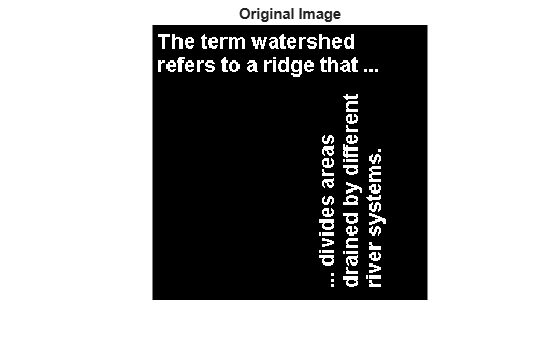Use filtering to create a second image that contains only those regions in the original image that do not have holes. For these regions, the Euler number property is equal to 1. Display filtered image.

```BW2 = bwpropfilt(BW,'EulerNumber',[1 1]); figure imshow(BW2) title('Regions with Euler Number == 1')```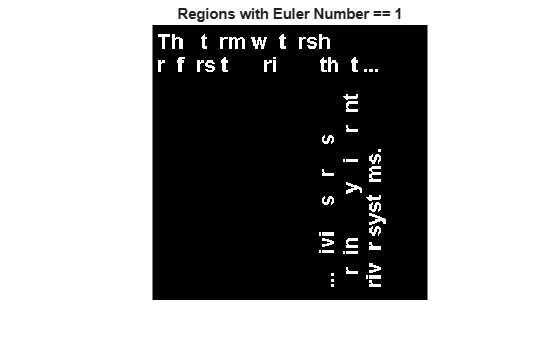`BW = imread('text.png');`

Find the ten objects in the image with the largest perimeters and display filtered image.

```BW2 = bwpropfilt(BW,'perimeter',10); figure; imshow(BW2) title('Objects with the Largest Perimeters')```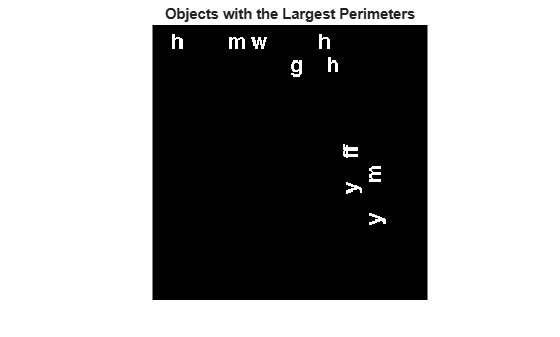## Input Arguments

collapse all

Image to be filtered, specified as a binary image.

Data Types: `logical`

Name of property on which to filter, specified as one of these values.

Pixel Value Measurements

Property NameDescription
`"Area"`Number of pixels in the region.
`"ConvexArea"`Number of pixels in the convex hull. The convex hull is the smallest convex polygon that can contain the region. For more information about classifying pixels on the boundary of the hull, see Classify Pixels That Are Partially Enclosed by ROI.
`"Eccentricity"`Eccentricity of the ellipse that has the same second-moments as the region. The eccentricity is the ratio of the distance between the foci of the ellipse and its major axis length. Values are numbers in the range [0, 1]. (0 and 1 are degenerate cases. An ellipse whose eccentricity is 0 is actually a circle, while an ellipse whose eccentricity is 1 is a line segment.)
`"EquivDiameter"`Diameter (in pixels) of a circle with the same area as the region, calculated as `sqrt(4*Area/pi)`.
`"EulerNumber"`Euler number (also known as the Euler characteristic), calculated as 1 minus the number of holes in the region.
`"Extent"`Ratio of pixels in the region to pixels in the total bounding box, calculated as `Area` divided by the area of the bounding box.
`"FilledArea"`Number of pixels in the region after filling all holes in the region.
`"MajorAxisLength"`Length (in pixels) of the major axis of the ellipse that has the same normalized second central moments as the region.
`"MinorAxisLength"`Length (in pixels) of the minor axis of the ellipse that has the same normalized second central moments as the region.
`"Orientation"`

Angle (in degrees) between the x-axis and the major axis of the ellipse that has the same second-moments as the region. The value is in the range (–90, 90].

This figure illustrates the axes and orientation of the ellipse. The left side of the figure shows an image region and its corresponding ellipse. The right side shows the same ellipse with the solid blue lines representing the axes. The red dots are the foci. The orientation is the angle between the horizontal dotted line and the major axis.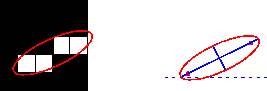`"Perimeter"`

Distance (in pixels) around the boundary of the region, calculated by adding the distance between each adjoining pair of pixels around the border of the region. This figure illustrates the pixels included in the perimeter calculation for a sample region.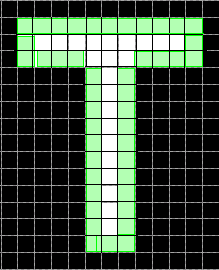`"Solidity"`Proportion of the pixels in the convex hull that are also in the region, calculated as `Area/ConvexArea`.

You can specify a pixel value measurement property from this table when you include a marker image, `I`, as an input argument to the function.

Pixel Value Measurements

Property Name Description
`"MaxIntensity"`Value of the pixel with the greatest intensity in the region.
`"MeanIntensity"`Mean of all the intensity values in the region.
`"MinIntensity"`Value of the pixel with the lowest intensity in the region.

Data Types: `char` | `string`

Minimum and maximum property values, specified as a 1-by-2 numeric vector of the form [low high]. Values must be nondecreasing.

Data Types: `single` | `double` | `int8` | `int16` | `int32` | `int64` | `uint8` | `uint16` | `uint32` | `uint64`

Number of objects to return, specified as a positive integer.

Data Types: `double`

Objects to retain, specified as `"largest"` or `"smallest"`.

Data Types: `char` | `string`

Marker image, specified as a grayscale image of the same size as the input binary image. Intensity values in the grayscale image define regions in the input binary image.

Data Types: `single` | `double` | `int8` | `int16` | `int32` | `int64` | `uint8` | `uint16` | `uint32` | `uint64`

Pixel connectivity, specified as one of these values.

Value

Meaning

Two-Dimensional Connectivities

`4`

Pixels are connected if their edges touch. Two adjoining pixels are part of the same object if they are both on and are connected along the horizontal or vertical direction.Current pixel is shown in gray.

`8`

Pixels are connected if their edges or corners touch. Two adjoining pixels are part of the same object if they are both on and are connected along the horizontal, vertical, or diagonal direction.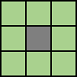Current pixel is shown in gray.

Connectivity can also be defined in a more general way by specifying a 3-by-3 matrix of `0`s and `1`s. The `1`-valued elements define neighborhood locations relative to the center element of `conn`. The matrix must be symmetric about its center element.

Data Types: `double` | `logical`

## Output Arguments

collapse all

Filtered image, returned as a binary image of the same size as `BW`.

## Version History

Introduced in R2014b